Question

# Consider the discrete-time periodic signal n- 2 (a) Determine the Discrete-Time Fourier Series (DTFS) coefficients ak...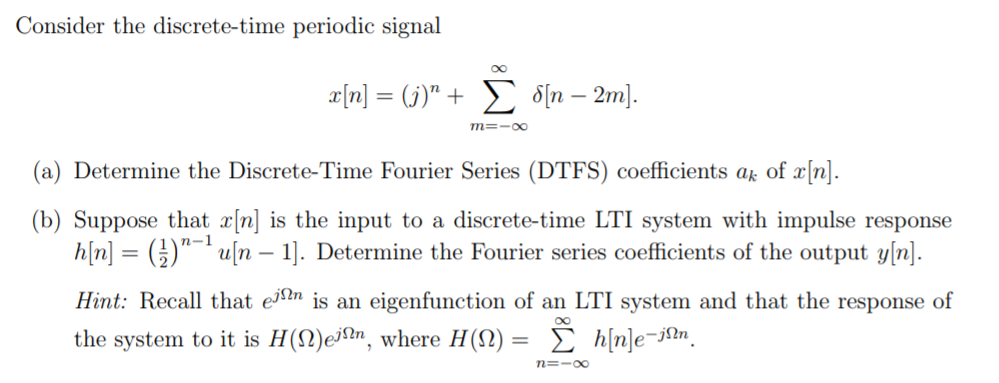Consider the discrete-time periodic signal n- 2 (a) Determine the Discrete-Time Fourier Series (DTFS) coefficients ak of X[n]. (b) Suppose that x[n] is the input to a discrete-time LTI system with impulse response hnuln - ]. Determine the Fourier series coefficients of the output yn. Hint: Recall that ejIn s an eigenfunction of an LTI system and that the response of the system to it is H(Q)ejfhn, where H(Q)-? h[n]e-jin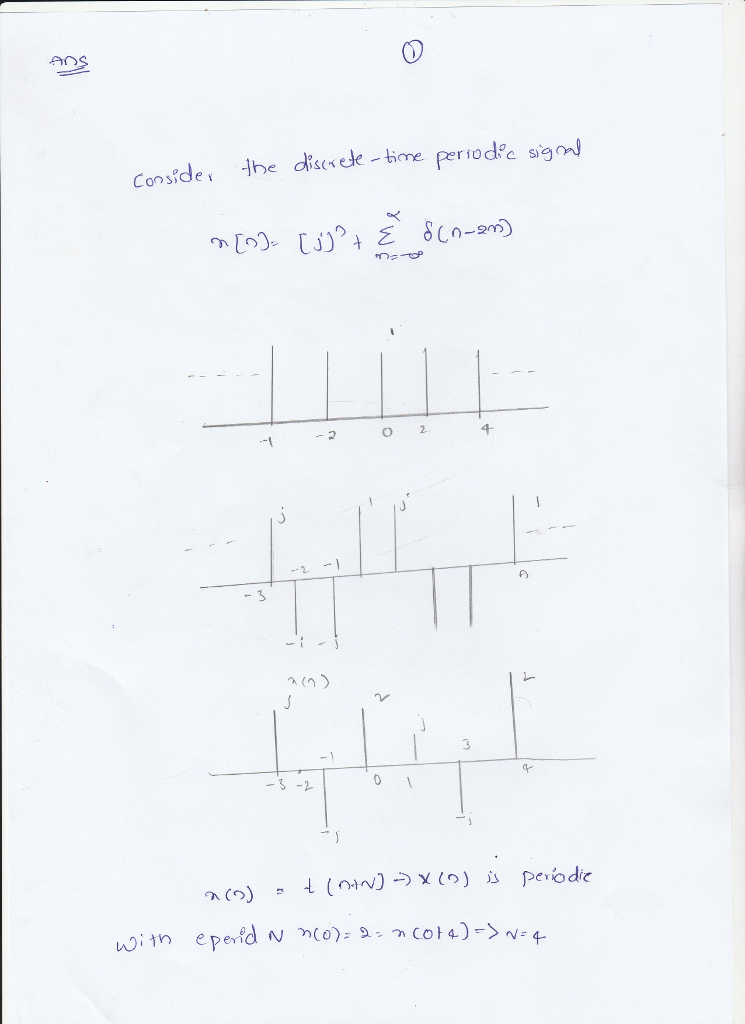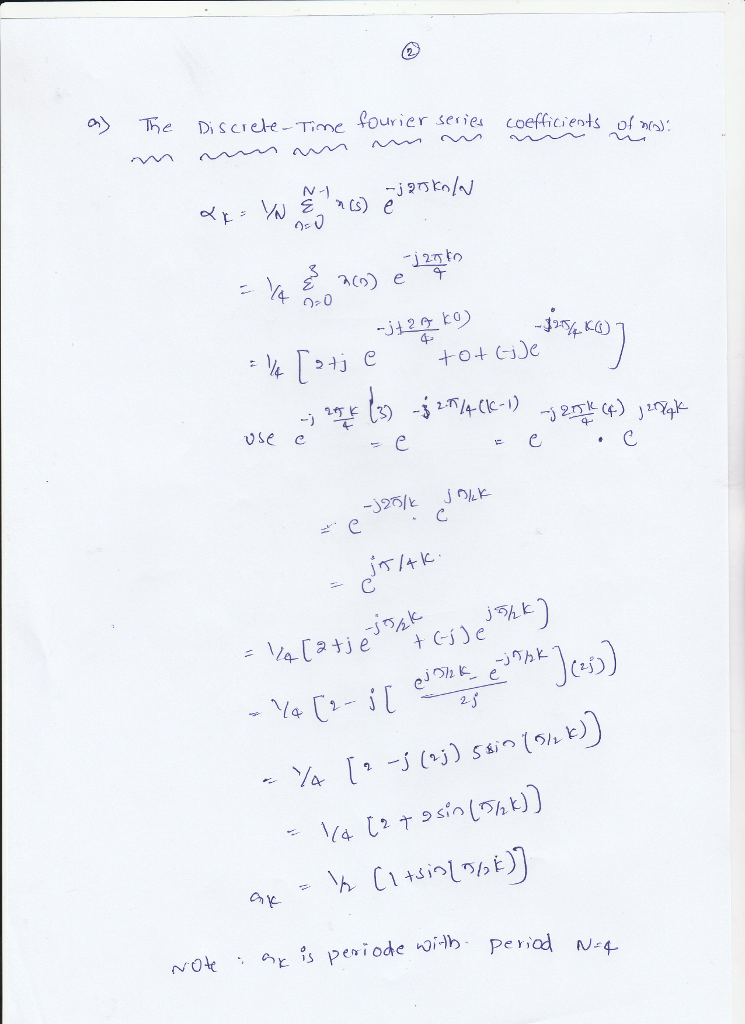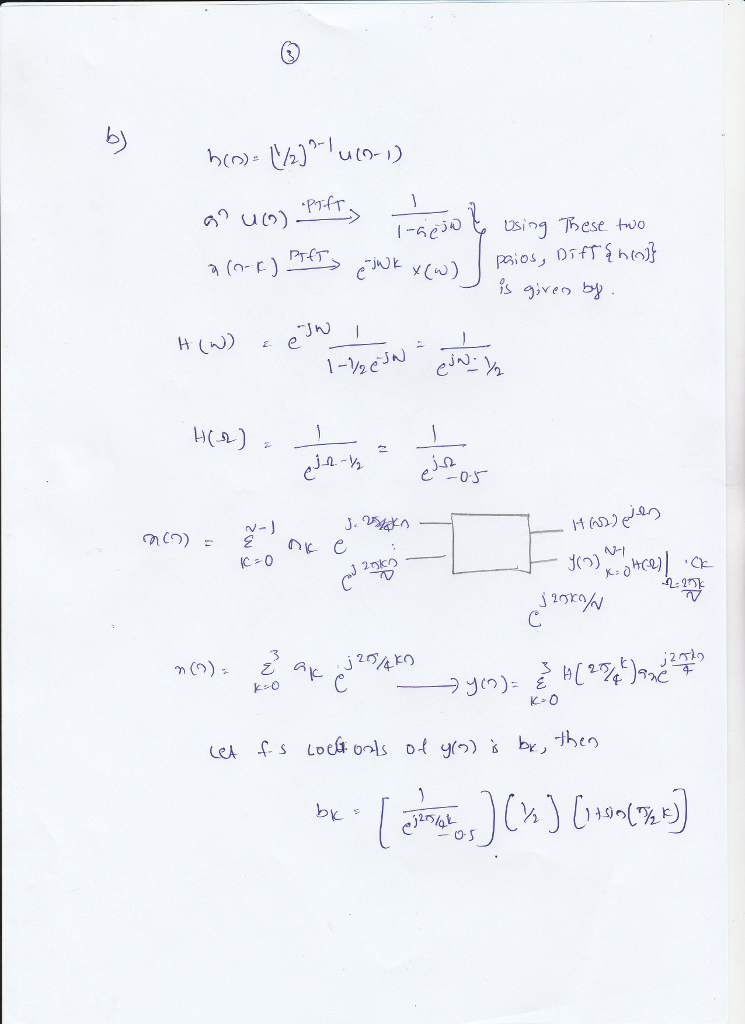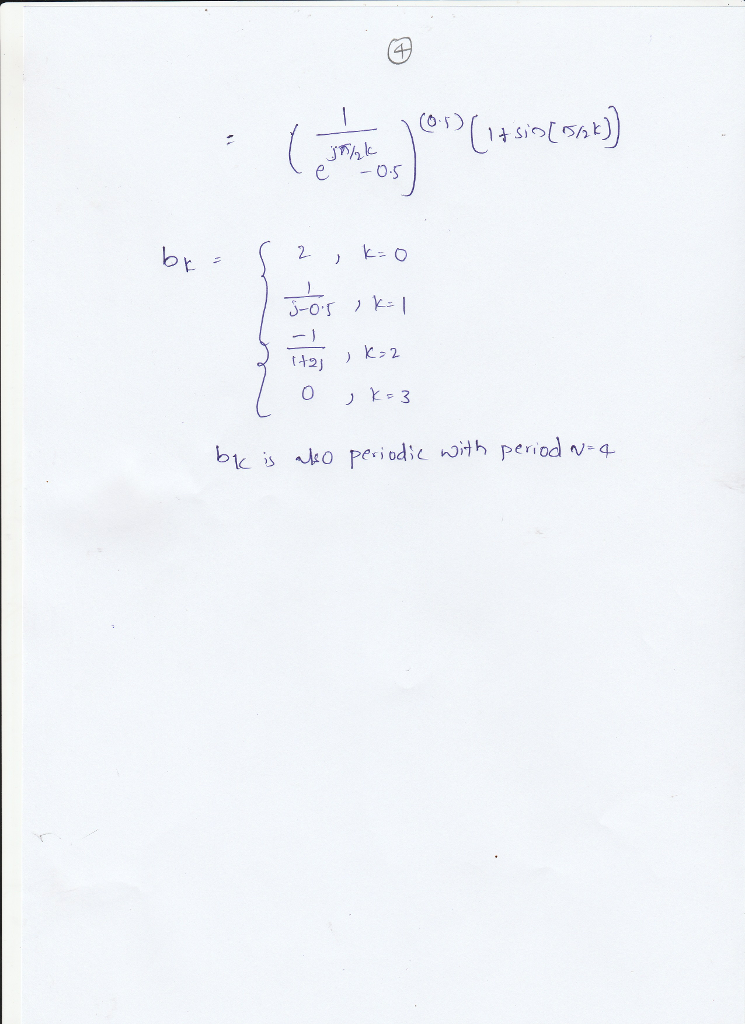#### Earn Coins

Coins can be redeemed for fabulous gifts.

Similar Homework Help Questions
• ### Prob. 2 Discrete-Time Fourier Series (DTFs) (a) A periodic signal, rin] is shown below. Use the...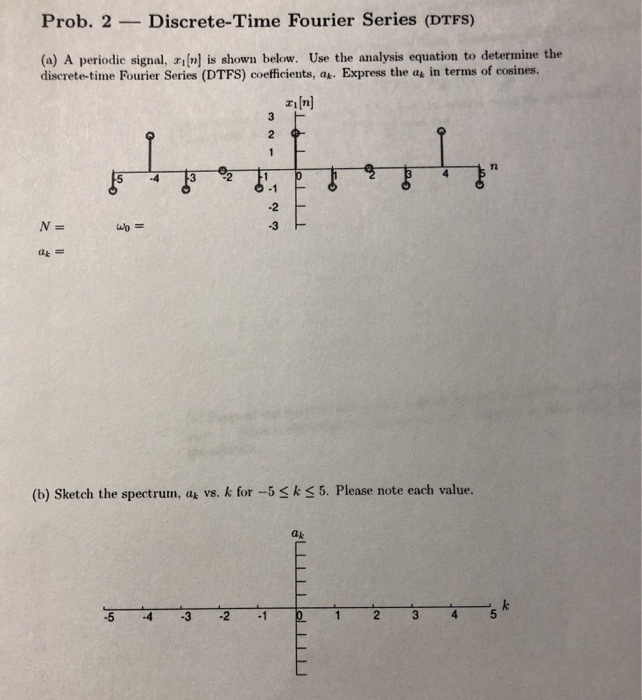Prob. 2 Discrete-Time Fourier Series (DTFs) (a) A periodic signal, rin] is shown below. Use the analysis equation to determine the discrete-time Fourier Series (DTFS) coefficients, a. Express the a in terms of cosines  -2 N= -3 (b) Sketch the spectrum, as vs. k for -5Sk s5. Please note each value. ak 2 5 Prob. 2 (cont.) -Discrete-Time Fourier Series (CTFS) (c) A periodic signal rafnl is given below. a2In] 2 1 E -3 what is the fundamental period...

• ### Problem 1 You are given the discrete-time LTI system with impulse response, Calculate the Fourier series coefficients o...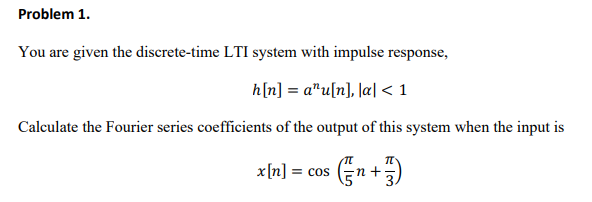Problem 1 You are given the discrete-time LTI system with impulse response, Calculate the Fourier series coefficients of the output of this system when the input is x[n] = cos(n+π) Problem 1 You are given the discrete-time LTI system with impulse response, Calculate the Fourier series coefficients of the output of this system when the input is x[n] = cos(n+π)

• ### Objective Conduct DTFT, DTFS on a periodic discrete signal. Task: Consider the system with impulse response...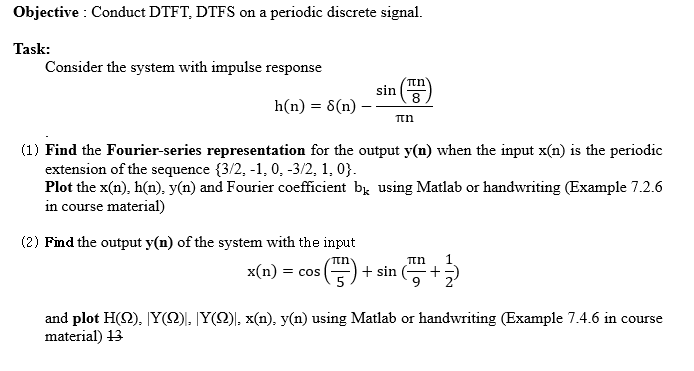Objective Conduct DTFT, DTFS on a periodic discrete signal. Task: Consider the system with impulse response Tth sin 8 h(n) S(n) Tn (1) Find the Fourier-series representation for the output y(n) when the input x(n) is the periodic extension of the sequence 3/2, -1,0, -3/2, 1,0 Plot the x(n), h(n), y(n) and Fourier coefficient bk using Matlab or handwriting (Example 7.2.6 irse material) in cour (2) Find the output y(n) of the system with the input 1 Tn Tn x(п)...

• ### Objective Conduct DTFT, DTFS on a periodic discrete signal. Task: Consider the system with impulse response...Objective Conduct DTFT, DTFS on a periodic discrete signal. Task: Consider the system with impulse response Tth sin 8 h(n) S(n) Tn (1) Find the Fourier-series representation for the output y(n) when the input x(n) is the periodic extension of the sequence 3/2, -1,0, -3/2, 1,0 Plot the x(n), h(n), y(n) and Fourier coefficient bk using Matlab or handwriting (Example 7.2.6 irse material) in cour (2) Find the output y(n) of the system with the input 1 Tn Tn x(п)...

• ### Solution required in MATLAB 1. Convolution and Discrete-Time Fourier Series (DTFS) (a) Generate a periodic signal r2[n]...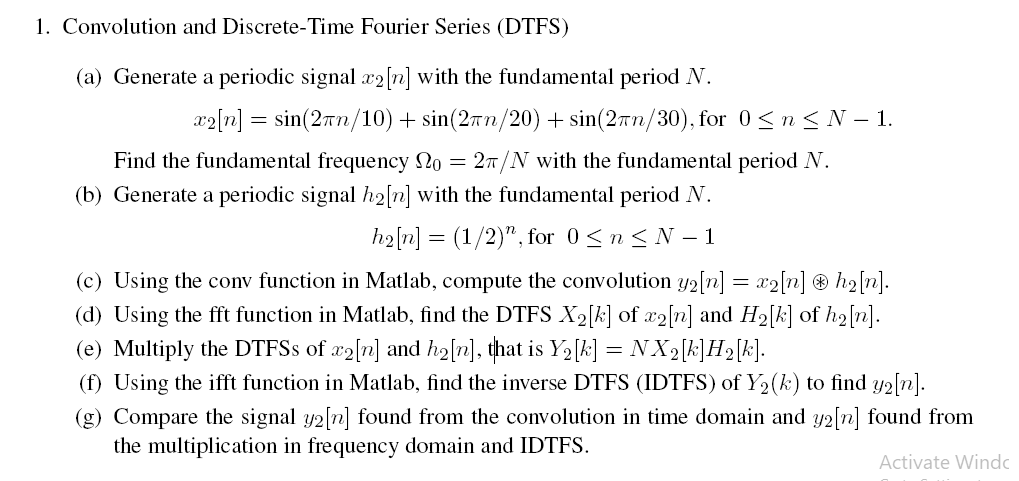Solution required in MATLAB 1. Convolution and Discrete-Time Fourier Series (DTFS) (a) Generate a periodic signal r2[n] with the fundamental period N ralla-sin(2nn/ İ0) + sin(2m, 2 ) + sin(2nn/30) for 0 < n < N-1 Find the fundamental frequency Ω0-2, N, with the fundamental period N. (b) Generate a periodic signal h2[n] with the fundamental period N haln] = (1/2)", for 0 < n < N-1 (e) Using the com ftuction n Matab, compute the compvolution (d) Using the...

• ### d)  The figure below shows the Fourier series coefficients ak of the DT periodic signal...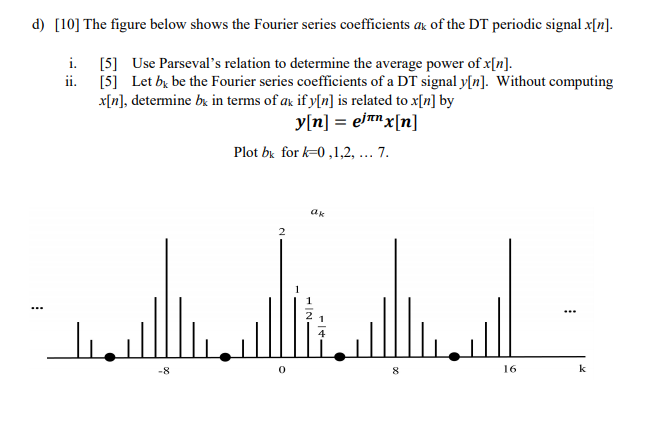d)  The figure below shows the Fourier series coefficients ak of the DT periodic signal x[n]. i. ii.  Use Parseval's relation to determine the average power of x[n].  Let bx be the Fourier series coefficients of a DT signal y[n). Without computing x[n], determine bk in terms of ak if y[n] is related to x[n] by y[n] = ejinx[n] Plot bk for k=0,1,2, ... 7. ak 16

• ### 6- A contiuous-time periodic signal x(t) is given graphically below. (a) Determine the exponential Fourier coefficients...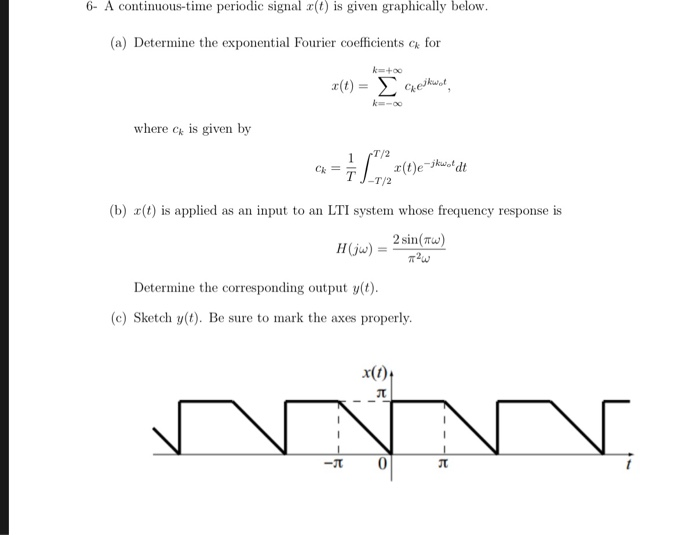6- A contiuous-time periodic signal x(t) is given graphically below. (a) Determine the exponential Fourier coefficients for k+oo a ()-ΣGeko, k-oo where c is given by T/2 1 (t)ek dt J-T/2 Ck= T (b) r(t) is applied as an input to an LTI system whose frequency response is H(ju)=2 sin(w Determine the corresponding output y(t) (e) Sketch y(t). Be sure to mark the axes properly -JT 6- A contiuous-time periodic signal x(t) is given graphically below. (a) Determine the exponential...

• ### 2.For the periodic DT signal shown in Top, a) determine the Fourier Series Coefficients. b) Use...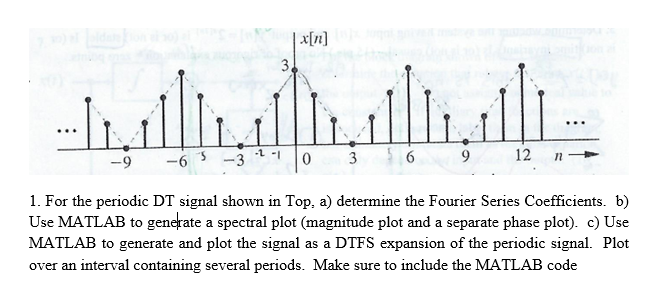2.For the periodic DT signal shown in Top, a) determine the Fourier Series Coefficients. b) Use MATLAB to generate a spectral plot (magnitude plot and a separate phase plot). c) Use MATLAB to generate and plot the signal as a DTFS expansion of the periodic signal. Plot over an interval containing several periods. Make sure to include the MATLAB code x[ri] -9 63 3 9 12 n 1. For the periodic DT signal shown in Top, a) determine the Fourier...

• ### 3. Find the discrete-time Fourier series (DTFS) and the corresponding spectra D, and ZD, for the...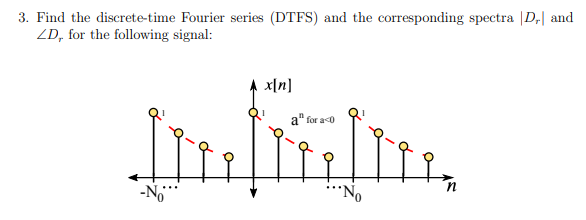3. Find the discrete-time Fourier series (DTFS) and the corresponding spectra D, and ZD, for the following signal: x[n] q -o- Q a" for 20 Ri Q -Q-Q mi - q Q

• ### 6- A continuous-time periodic signal r(t) is given graphically below (a) Determine the exponential Fourier coefficients...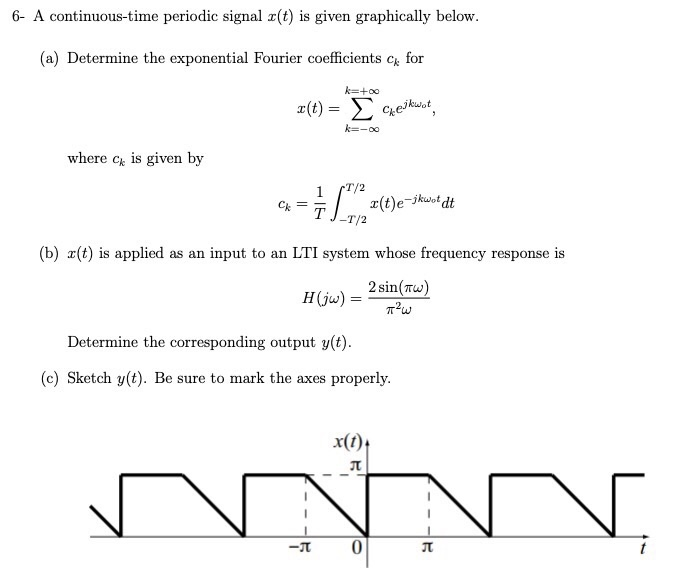6- A continuous-time periodic signal r(t) is given graphically below (a) Determine the exponential Fourier coefficients c for k+oo r(t) Cet k=-oo where ck is given by T/2 x(t)ejkwotdt -T/2 Ск T (b) (t) is applied as an input to an LTI system whose frequency response is H(jw)2 sin(w) = Determine the corresponding output y(t) (c) Sketch y(t). Be re to mark the ces properly su x(t)t 0 -T 6- A continuous-time periodic signal r(t) is given graphically below (a)...